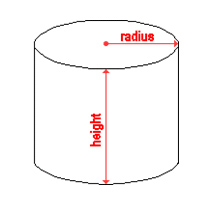ALL
MyHealth.Alberta.ca Network
Cleaning a Cistern

# Water Safety

## Cleaning a Cistern

### ​​​​How do I clean my cistern?

1. Make sure your footwear and equipment are clean before you go in the cistern (follow confined space entry precautions). Drain the cistern completely. Don’t use a sewage hauler to pump out the cistern.
2. Wash all inside surfaces of the cistern. Use a pressure washer or stiff brush and a non-corrosive, non-foaming, food-grade detergent to loosen debris and sediment. If needed, remove bottom sludge with a wet-dry vacuum.
3. Rinse the cistern with clean, potable water, and then drain the water to waste.
4. After cleaning and rinsing, the cistern is ready to disinfect (see instructions below).

### ​How much disinfectant do I need to use?

How much disinfectant you need depends on how much water the cistern holds. If you don’t have this information, you can estimate by using the ways shown below.Box Cistern
To estimate how much water your box cistern (see Figure 1) can hold, you’ll need a calculator and you’ll need to know these measurements (in metres):

• the length (L)
• the width (W)
• the height (H) (this is the maximum depth of water)

Now, take these measurements and multiply them by each other (L x W x H). This will give you a number that you need to multiply by 1000. The answer will tell you how many litres of water your cistern can hold, so you can figure out how much disinfectant to use.

 Example: If your cistern has these measurements (length= 2.5 m, width= 1.5 m, and height= 1.7 m), this is how you calculate how much water it would hold.2.5 x 1.5 x 1.7 = 6.38​ m36.38​ x 1000 = 638​0 litres​Cylindrical Cistern
To estimate how much water your cylindrical cistern (see Figure 2) can hold, you’ll need a calculator and you’ll need to know these measurements (in metres):

• height

Now, take the measurements and follow these instructions:

1. Take the radius and multiply it by itself.
2. Take this number and multiply the number by 3.14.
3. Now, take this number and multiply it by the height.
4. Take this number and multiply it by 1000.

The answer will tell you how many litres of water your cistern can hold, so you can figure out how much disinfectant to use.

 Example: If your cistern has these measurements (radius = 1.1 m and height= 1.8 m), this is how you calculate how much water it would hold.1.1 x 1.1 = 1.211.21 x 3.14 = 3.83.8 x 1.8 = 6.84 6.84 x 1000 = 6840 litres​

Current as of: March 13, 2018

Author: Environmental Public Health, Alberta Health Services